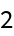#Function Repository Resource:

# ArgumentCount

Count the number of arguments a pure function or compiled function takes

Contributed by: Sjoerd Smit
 ResourceFunction["ArgumentCount"][Function[…]] counts how many arguments the given function takes.

## Details and Options

ResourceFunction["ArgumentCount"] works with CompiledFunction and CompiledCodeFunction.
If a Function uses SlotSequence, the returned argument count will be ∞ unless the option "IgnoreSlotSequence" True is set.

## Examples

### Basic Examples

Find the highest numbered slot in a function:

 In:=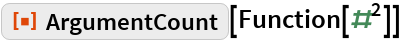Out=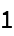In:=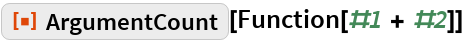Out=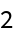In:=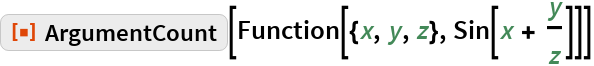Out=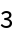In this function, slot 2 goes unused, but it will still be counted:

 In:=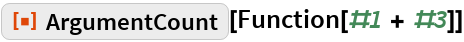Out=### Scope

Slots in inner functions will not be counted:

 In:=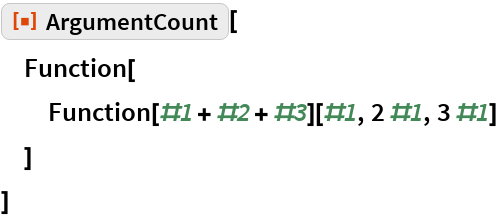Out=A function that uses SlotSequence will be counted as having infinite arguments:

 In:=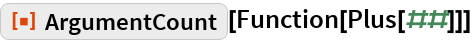Out=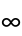String slots will be counted as being part of the first argument:

 In:=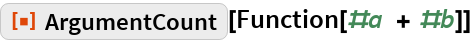Out=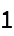In:=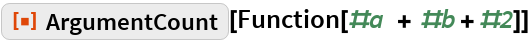Out=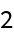ArgumentCount works with compiled functions:

 In:=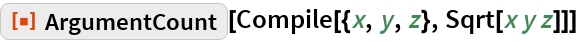Out=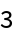### Options

#### IgnoreSlotSequence

A function that uses SlotSequence will be counted as having infinite arguments:

 In:=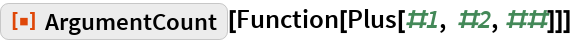Out=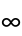This behavior can be changed with the "IgnoreSlotSequence" option:

 In:=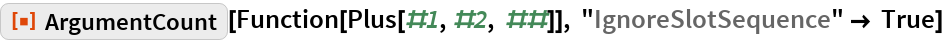Out=